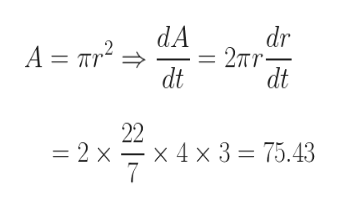The radius of a circle is increasing at the rate of 3 centimeters per second. At the instant the radius is 4cm, find a.) the rate of change of the area of the circle. B.) the rate of change of the circumference of the circle.

Question

The radius of a circle is increasing at the rate of 3 centimeters per second. At the instant the radius is 4cm, find a.) the rate of change of the area of the circle. B.) the rate of change of the circumference of the circle.

Step 1

If r is the radius of he circle, then

Area of the Circle, A = πr2

Circumference of the circle, C = 2πr

dr/dt = 3 centimeters per second

At a particular instant of time, r = 4 cm

Step 2

Part (a)

Please see the white board. The rate of change of th...help_outlineImage Transcriptionclosedr 2Tr dt dA A r2 dt 22 x 4 x 3 75.43 =2 x fullscreen

Want to see the full answer?

See Solution

Want to see this answer and more?

Our solutions are written by experts, many with advanced degrees, and available 24/7

See Solution
Tagged in

Derivative